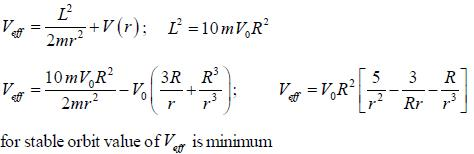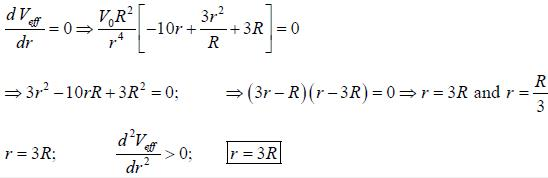# Test: Classical Mechanics - 1

## 20 Questions MCQ Test GATE Physics Mock Test Series | Test: Classical Mechanics - 1

Description
This mock test of Test: Classical Mechanics - 1 for GATE helps you for every GATE entrance exam. This contains 20 Multiple Choice Questions for GATE Test: Classical Mechanics - 1 (mcq) to study with solutions a complete question bank. The solved questions answers in this Test: Classical Mechanics - 1 quiz give you a good mix of easy questions and tough questions. GATE students definitely take this Test: Classical Mechanics - 1 exercise for a better result in the exam. You can find other Test: Classical Mechanics - 1 extra questions, long questions & short questions for GATE on EduRev as well by searching above.
QUESTION: 1

### Consider a satellite in a circular orbit around the earth. Then properties of the satellite depend on the radius of the orbit r  as follows: speed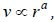time period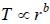angular momentum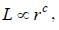and kinetic energy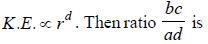Solution:

For a satellite in a circular orbit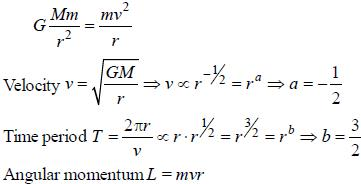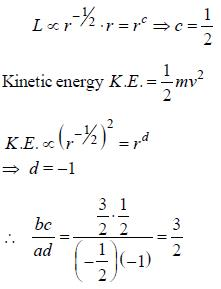QUESTION: 2

Solution: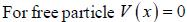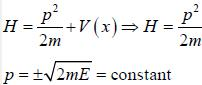QUESTION: 3

### For the Lagrangian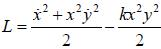find equation of motion

Solution:

Explanation : L = (x2+ x,2y2)/2 - kx,2y2/2

d/dt(dL/dx) - dL/dx = 0

=> d/dt(x) - xy2 + kxy2 = 0

=> x = xy2 - kxy2

d/dt(dL/dy) - dL/dy = 0

d/dt(x2y) + kx2y = 0

=> x2y + 2xy + kx2y = 0

xy = -2xy - kxy

QUESTION: 4

The value of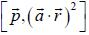is

Solution:

From the Poisson's bracket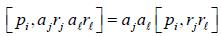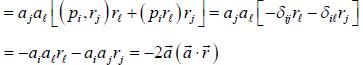QUESTION: 5

A bullet is fired horizontally in the north direction with a speed u at θ latitude. It hits a target 1 meter away. Calculate the Coriolis acceleration.

Solution: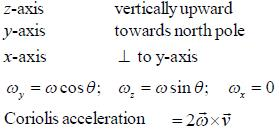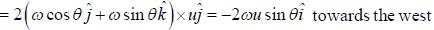*Answer can only contain numeric values
QUESTION: 6

If a spring of spring constant k is stretched by x0, the work done is W0. Now, a second spring of spring constant 3k is stretched by x0/3. The work done is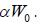The value of a is _____ (Correct upto one decimal place).

Solution:

For first spring,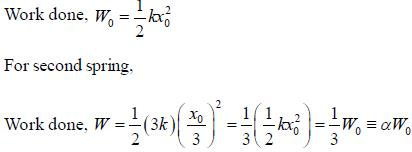∴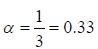*Answer can only contain numeric values
QUESTION: 7

The moment of inertia of pairs of solid sphere each having mass m radius r kept in contact about a tangent passing through the point of contact is βmr2. The value of β is ______ . (Upto one decimal place)

Solution: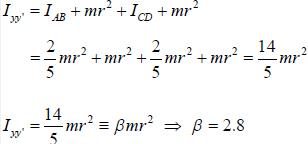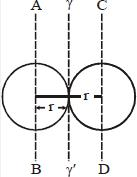*Answer can only contain numeric values
QUESTION: 8

The moment of inertia tensor of a rigid body is given by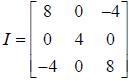Moment of inertia about an axis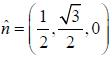is _______.

Solution: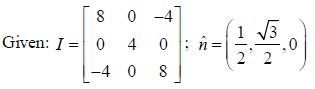Moment of inertia about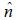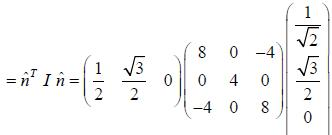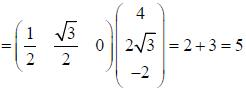*Answer can only contain numeric values
QUESTION: 9

A uniform chain of length L and mass M is lying on a smooth table and one third of its length is hanging vertically down over the edge of the table. The work required to pull the hanging part on the table is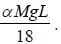The value of α is _________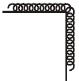Solution: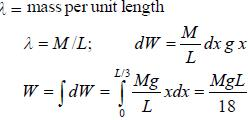QUESTION: 10

The Hamiltonian of a system is given by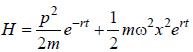It describes the motion of

Solution:

Equations of motion are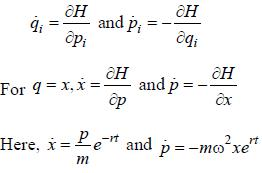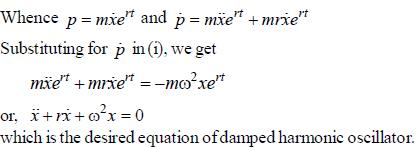*Answer can only contain numeric values
QUESTION: 11

The muon is an unstable particle that spontaneously decay into an electron and two neutrinos if the number of muons at t = 0 is N0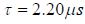is the mean life time of muon. Suppose muons move at speed 0.95c, the number of muons remain after travelling a distance of 3.0 km is _______ (in terms of N0) (upto three decimal places)

Solution:

S frome attached to the earth and S'frome to be rest frome of muon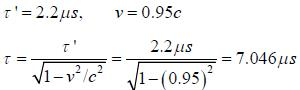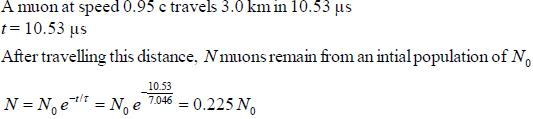*Answer can only contain numeric values
QUESTION: 12

A comet in an orbit about the sun has a velocity 10km/sec at aphelion and 80km/sec at perihelion if the earth's velocity in a circular orbit is 30 km/sec and the radius of its orbit is 1.5 x 108km, the aphelion distance Ra for comet is ____ x 108km. (Nearest integer)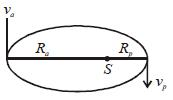Solution:

Let v be velocity of the earth, R the radius of the earth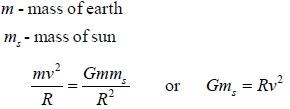applying conservation of energy and the angular momentum of comet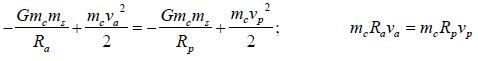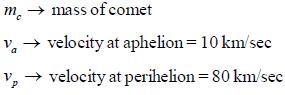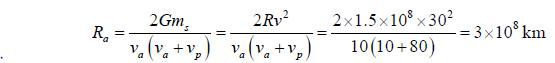QUESTION: 13

Find Lagrangian of the pendulum of mass m and length R attached to a spring, the other end of which is fixed at the bottom as shown in the figure. The length of the undeformed spring is l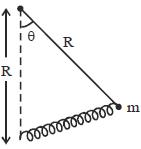Solution:

Lagrangian, L = T - V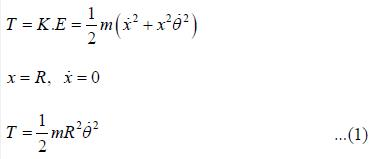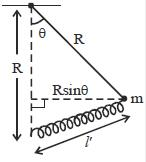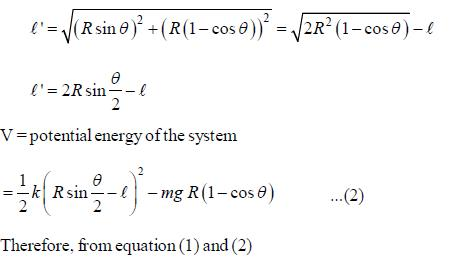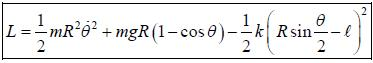QUESTION: 14

The kinetic energy  of a particle in terms of coordinate r and q = sin θ, where r & θ are polar coordinate is

Solution:

Kinetic energy of particle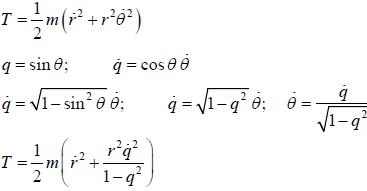QUESTION: 15

Consider the At wood's machine. Let x and y be the vertical position of the middle mass and right mass, respectively with upward  taken to be positive. The  conjugate momenta, Px and Py respectively,  are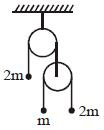Solution:

If the right two masses move up by x and y then the left mass move down by (x+y)/2
∴ Lagrangian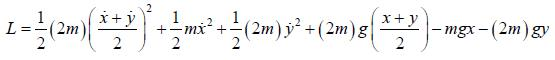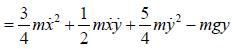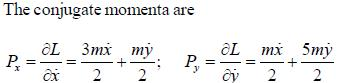QUESTION: 16

If Iij is the tensor of inertia of a solid sphere, x2+y2+z2 = a2, of mass M in the first octant, then

Solution:

In first quadrant,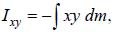dm = pdx dy dz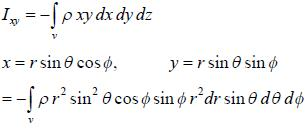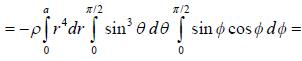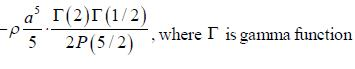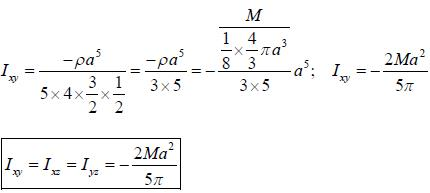QUESTION: 17

A train with proper length L moves with speed 5c/13 with respect to the ground. A ball is thrown from the back of the train to the front. The speed of the ball with respect to the train is c/3. As viewed by someone on the ground how much time does the ball spend in the air and how far does it travel.

Solution: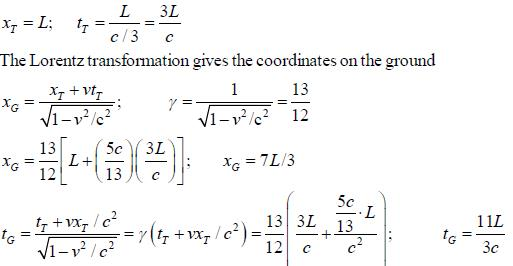QUESTION: 18

The force of interaction between a particle of mass m1 and a second particle of mass m2 separated by a distance r is given by an attractive gravitational force and a repulsive force that is proportional to r-3, with probability constant C,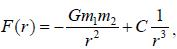the angular frequency of small oscillation about the stable equilibrium position is

Solution: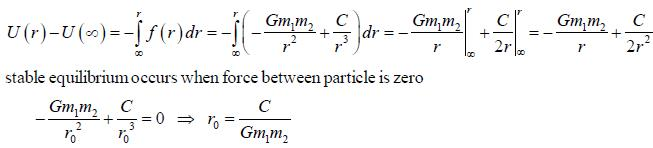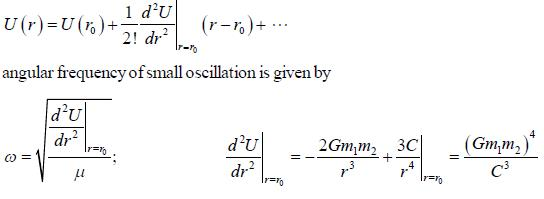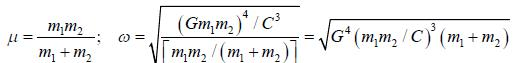*Answer can only contain numeric values
QUESTION: 19

A disk of mass m and radius R is attached to a spring of constant K as shown in the figure. The disk rolls and forth without slipping. The angular frequency of the motion of the disk is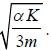The value of α is ______.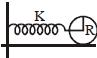Solution: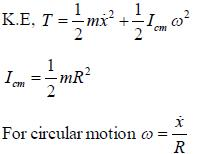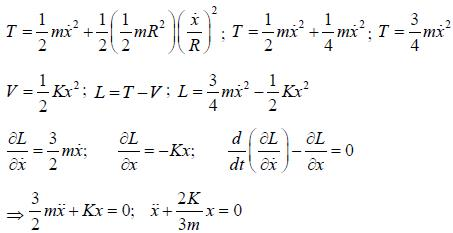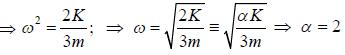*Answer can only contain numeric values
QUESTION: 20

The particle of mass m and angular momentum L2 = 10mV0R2 moves in a potential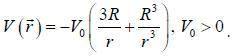The radius of the stable circular orbit is _____R.

Solution: